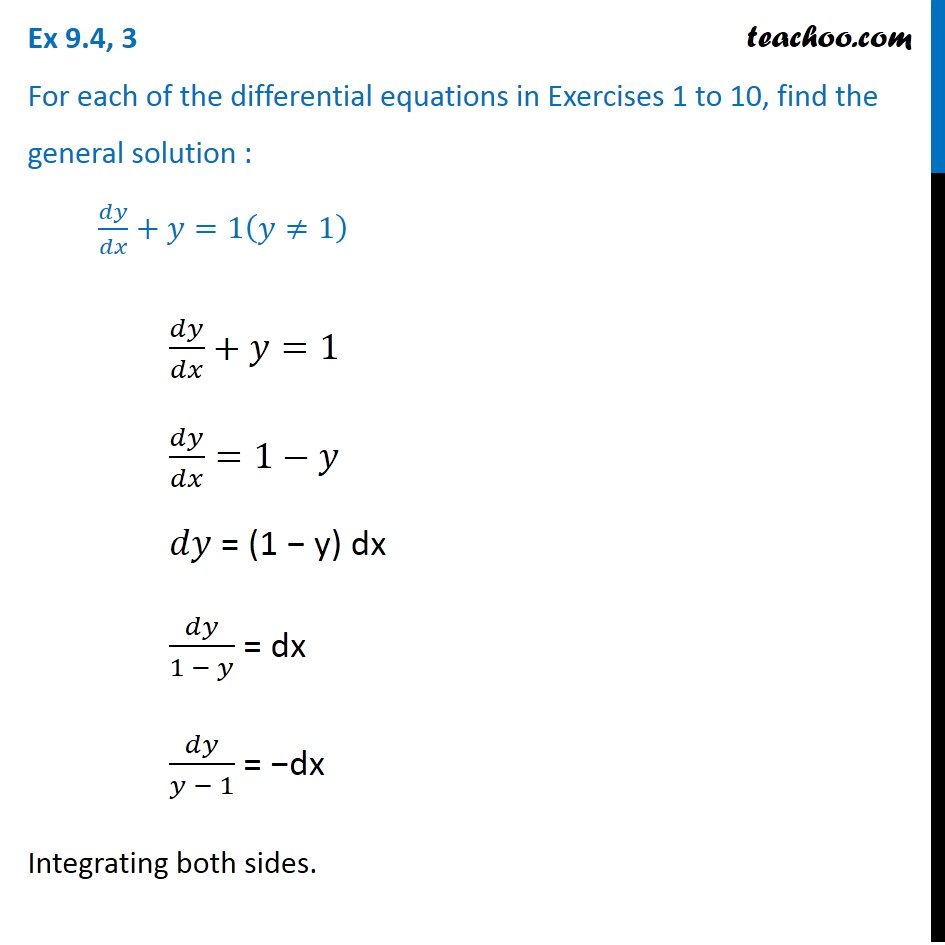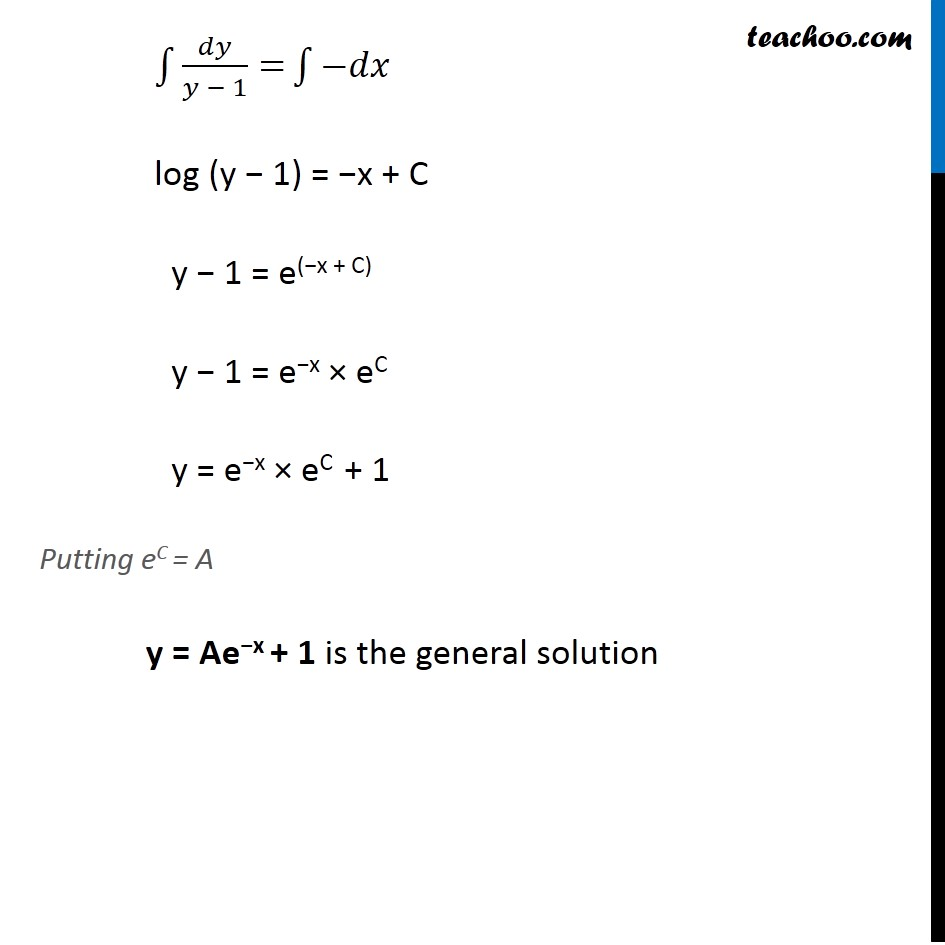Ex 9.3

Chapter 9 Class 12 Differential Equations
Serial order wiseLearn in your speed, with individual attention - Teachoo Maths 1-on-1 Class

### Transcript

Ex 9.3, 3 For each of the differential equations in Exercises 1 to 10, find the general solution : 𝑑𝑦/𝑑𝑥+𝑦=1(𝑦≠1) 𝑑𝑦/𝑑𝑥+𝑦=1 𝑑𝑦/𝑑𝑥=1−𝑦 𝑑𝑦 = (1 − y) dx 𝑑𝑦/(1 − 𝑦) = dx 𝑑𝑦/(𝑦 − 1) = −dx Integrating both sides. ∫1▒〖𝑑𝑦/(𝑦 − 1)=〗 ∫1▒〖−𝑑𝑥〗 log (y − 1) = −x + C y − 1 = e(−x + C) y − 1 = e−x × eC y = e−x × eC + 1 Putting eC = A y = Ae−x + 1 is the general solution